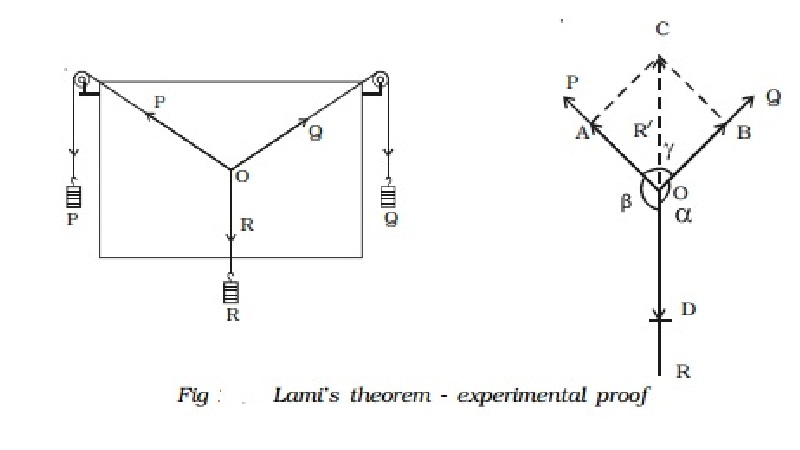Home | | Physics | | Physics | Experimental verification of triangle law, parallelogram law and Lami's theorem

Experimental verification of triangle law, parallelogram law and Lami's theoremTwo smooth small pulleys are fixed, one each at the top corners of a drawing board kept vertically on a wall as shown in Fig.. The pulleys should move freely without any friction.

Experimental verification of triangle law, parallelogram law and Lami's theorem

Two smooth small pulleys are fixed, one each at the top corners of a drawing board kept vertically

on a wall as shown in Fig.. The pulleys should move freely without any friction. A light string is made to pass over both the pulleys. Two slotted weights P and Q (of the order of 50 g) are taken and are tied to the two free ends of the string. Another short string is tied to the centre of the first string at O. A third slotted weight R is attached to the free end of the short string. The weights P, Q and R are adjusted such that the system is at rest.The point O is in equilibrium under the action of the three forces P, Q and R acting along the strings. Now, a sheet of white paper is held just behind the string without touching them. The common knot O and the directions of OA, OB and OD are marked to represent in magnitude, the three forces P, Q and R on any convenient scale (like 50 g = 1 cm).

The experiment is repeated for different values of P, Q and R and the values are tabulated.

To verify parallelogram law

To determine the resultant of two forces P and Q, a parallelogram OACB is completed, taking OA representing P, OB representing Q and the diagonal OC gives the resultant. The length of the diagonal OC and the angle COD are measured and tabulated (Table).

OC is the resultant R of P and Q. Since O is at rest, this resultant R must be equal to the third force R (equilibrant) which acts in the opposite direction. OC = OD. Also, both OC and OD are acting in the opposite direction. COD must be equal to 180o.

If OC = OD and COD = 180o, one can say that parallelogram law of force is verified experimentally.

Verification of parallelogram lawS.No. P       Q       R       OA    OB    OD    OC    COD

(R)     (R|)

1.

2.

3.

To verify Triangle Law

According to triangle law of forces, the resultant of P (= OA = BC) and Q (OB) is represented in magnitude and direction by OC which is taken in the reverse direction.

Alternatively, to verify the triangle law of forces, the ratios , P/OA, Q/OB and R/O C,  are calculated and are tabulated (Table). It will be found out that, all the three ratios are equal, which proves the triangle law of forces experimentally.Verification of triangle law

S.No. P       Q       R1     OA    OB    OC              P/OA          Q/OB       R'/OC

1.

2.

3.

To verify Lami's theorem

To verify Lami's theorem, the angles between the three forces, P, Q and R (i.e) BOD = αAOD = β and AOB = γ are measured using protractor and tabulated (Table 2.4). The ratios P/sin α, Q sin /β and R/sin γ

are calculated and it is found that all the three ratios are equal and this verifies the Lami's theorem.

Verification of Lami's theorem

S.No. P   Q R       α β γ           P/sinα   Q/sinβ  R/sinγ

1.

2.

3.Conditions of equilibrium of a rigid body acted upon by a system of concurrent forces in plane

If an object is in equilibrium under the action of three forces, the resultant of two forces must be equal and opposite to the third force. Thus, the line of action of the third force must pass through the point of intersection of the lines of action of the other two forces. In other words, the system of three coplanar forces in equilibrium, must obey parallelogram law, triangle law of forces and Lami's theorem. This condition ensures the absence of translational motion in the system.

The algebraic sum of the moments about any point must be equal to zero. Σ M = 0 (i.e) the sum of clockwise moments about any point must be equal to the sum of anticlockwise moments about the same point. This condition ensures, the absence of rotational motion.

Study Material, Lecturing Notes, Assignment, Reference, Wiki description explanation, brief detail

Related Topics